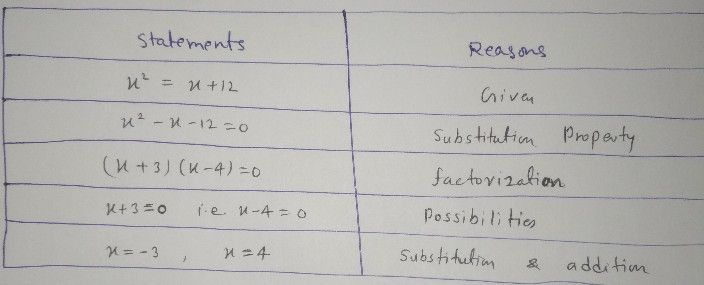Symbol
Problem$8.$ GIVEN: $x^{2}=x+12$ PROVE: $x=4orx=-3$ Statements Reasons